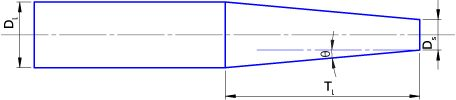# Taper Calculator

Created by Rahul Dhari
Reviewed by Steven Wooding
Last updated: Feb 15, 2022

The taper calculator deals with determining the parameters related to tapering a workpiece. A taper is like a conical frustum with two ends having different sizes. With modern automated manufacturing processes, manually adjusting the tool alignment might be obsolete. But you still need to plug in the numbers for the desired dimensions to make a tapered workpiece.

Drill chucks, arbors, nails, bolts, and screws are some of the common applications of tapers. Various parameters for tapering include length, diameters, and taper angle. Read on to understand what is taper and how to calculate taper per inch?

## What is taper?

Tapering is the process of machining a workpiece into a conical profile, i.e., reducing dimensions of one end gradually with respect to length. It is also comparable with a frustum or truncated cone. Considering a circular cross-section workpiece, the section at the larger end is called the major section and has the diameter as major diameter (Dl), whereas the smaller section is known as the minor section and the diameter is the minor diameter (Ds). The elements of taper are:

• Taper length (Tl): The distance between minor and major sections of the workpiece.
• Taper per inch (TPI): The reduction in the cross-sectional diameter per inch of length. For a workpiece having taper length, Tl, the taper per inch formula is:
TPI = (Dl - Ds) / Tl

The above formula is modified as the following equation to calculate taper per foot, TPF:

TPF = 12 * (Dl - Ds) / Tl
• Taper angle (θ): The angle between the center line and the sloped side. The taper angle is given by the equation:
θ = atan (TPI/2)

Tapers can be specified using three parameters:

• Major diameter;
• Minor diameter; and
• Taper length.

A typical taper can be specified using the above three dimensions. However, some other ways to mention a taper are following combinations of parameters.

• Major diameter, taper length, and taper per foot or inches.
• Major diameter, minor diameter, and end-to-end length.
• Major diameter, minor diameter, and taper angle.

## Types of taper

Some of the common types of tapers are classified based on:

• Size – Jarno, Metric, Brown and Sharpe, Morse, and Jacobs tapers.
• Location – Internal and external tapers.
• Use – Self-holding and quick releasing tapers.

## How to calculate taper per foot?

To calculate taper per foot:

1. Enter major diameter, Dl.
2. Fill in the minor diameter, Ds.
3. Insert taper length, Tl.
4. The taper angle calculator will return the value of taper per inch or foot and the taper angle.

## Example: Using the taper calculator

Estimate the taper length for the workpiece having major and minor diameters as 12 and 6 inches, respectively. Take taper angle as 80.5°.
The taper length is calculated as:

1. Enter major diameter, Dl = 12 in.
2. Fill in the minor diameter, Ds = 6 in.
3. Insert taper angle, θ = 80.5°.
4. The taper angle calculator will return the taper per inch.
TPI = 2 * tan θ = 2 * tan 80.5° = 11.952/in
1. Further, using the taper length formula:
Tl = (Dl - Ds) / TPI
Tl = (12 - 6) / 0.5 = 0.502 in

## Applications of tapers

Tapers are extensively used for plug-in adapters, mounting drill bits into chucks, toolholders, and self-holding circular objects.

## FAQ

### What is a taper?

Taper is a shape or profile where one end is smaller than the other and the reduction in cross-section is gradual.

### How do I find taper per inch?

To find taper per inch:

1. Find the difference between major and minor diameters.
2. Divide the difference with the length of the taper region.

### How do I estimate taper per foot?

1. Find the difference between major and minor diameters.
2. Divide the difference with the length of the taper region.
3. Multiply the taper per inch by 12.

### How do I calculate taper angle?

The taper angle is calculated using the inverse tangent function of half of the taper per inch.

Rahul DhariMajor diameter (Dₗ)
in
Minor diameter (Dₛ)
in
Length of taper (Tₗ)
in
Taper angle (θ)
deg
Taper (T)
/
in
People also viewed…

### Flat vs. round Earth

Omni's not-flat Earth calculator helps you perform three experiments that prove the world is round.

### Gallons per minute

Find out the water flow rate of your shower with this gallons per minute calculator (GPM).

### Sand

Use our sand calculator to determine the amount of sand you need and how much will it cost you.

### Social Media Time Alternatives

Check what you could have accomplished if you get out of your social media bubble.